# Important Questions for Class 6 Chapter 5 Mathematics## myCBSEguide

Trusted by 70 Lakh Students

Important Questions for Class 6 Chapter 5 Mathematics. myCBSEguide has just released Chapter Wise Question Answers for class 6 Maths. There chapter wise Practice Questions with complete solutions are available for download in myCBSEguide website and mobile app. These Extra Questions with solution are prepared by our team of expert teachers who are teaching grade in CBSE schools for years. There are around 4-5 set of solved Mathematics Extra questions from each and every chapter. The students will not miss any concept in these Chapter wise question that are specially designed to tackle Exam. We have taken care of every single concept given in CBSE Class 6 Mathematics syllabus and questions are framed as per the latest marking scheme and blue print issued by CBSE for Class 6.

CBSE Class 6 Maths Extra Questions

## CBSE Class 6 Maths Understanding Elementary Shapes Extra Questions

Ch – 5 Understanding Elementary Shapes

1. How many right angles do you make if you start facing south and turn clockwise to west?
1. 1
2. 2
3. 3
4. 4
2. Find the number of right angles turned through by the hour hand of a clock when it goes from 3 to 6.
1. 4
2. 2
3. 1
4. 3
3. What fraction of a clockwise revolution does the hour hand of a clock turn through, when it goes from 12 to 3?
1.  {tex}\frac{1}{3}{/tex}
2. 1
3. {tex}\frac{1}{2}{/tex}
4. {tex}\frac{1}{4}{/tex}
4. What is the angle name for half a revolution?
1. Right angle
2. Straight angle
3. Complete angle
4. Reflex angle
5. How do we write “{tex}\overrightarrow { \mathrm { PQ } }{/tex} is perpendicular to {tex}\overrightarrow { \mathrm { RS } }{/tex}” symbolically?
1.  {tex}\overleftrightarrow {PQ}//\overleftrightarrow {RS}{/tex}
2.  {tex}\overleftrightarrow {PQ} \ne \overleftrightarrow {RS}{/tex}
3.  {tex}\overleftrightarrow {PQ} \bot \overleftrightarrow {RS}{/tex}
4. {tex}\overleftrightarrow {PQ} = \overleftrightarrow {RS}{/tex}
6. Match the following 3D shape and its edges.

Column AColumn B
1. Cube(a) 6
2. Square pyramid(b) 12
3. Triangular prism(c) 8
4. Triangular pyramid(d) 9
7. Fill up the following:

1. Measure of a complete angle is ____________o.
2. The triangle in which ____________ sides are equal is called isosceles triangle.
3. Each of its angles rectangle measures ____________o.
4. A cube has ____________ vertices.
8. State true or false:

1. Sum of any two sides of a triangle is greater than the third side.
2. An equilateral triangle is also considered as an isosceles triangle
3. A polygon is regular if its all sides are equal.
4. Opposite faces of a cuboid are equal in size.
9. How many faces a tetrahedron have?
10. What is the angle name for half a revolution?
11. Draw a hexagon and write its sides and diagonals?
12. If B is the mid point of {tex}\overline{A C}{/tex} and C is the point of {tex}\overline{\mathrm{BD}}{/tex} . where A, B, C, D lie on a straight line, say why AB = CD?
13. Draw a rough sketch of a regular octagon. Draw a rectangle by joining exactly four of the vertices of the octagon.
14. Measure the angles given below, using the Protractor and write down the measure.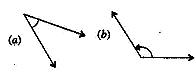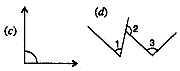15. All equilateral triangle are isosceles, but all isosceles triangle are not equilateral. Justify the statement.

Ch – 5 Understanding Elementary Shapes

1. 1
Explanation: The four main direction north, east, south, west. Each of them are at 90o clockwise, i.e. we have to move 90o to move north to east, another 90o from east to south like that.
So South to west we have to move only one 90o . so answer is 1
1. 1
Explanation: Hour hand move 360o from 12 to 12 . So it moves 3 hr from 3 to 6 . The factor of 3 to 12 {tex}= 3/12 = 1/4{/tex}
Right angle = 90o , factor of 90o with 360o {tex}=  90 /360 =1/4 .{/tex}
So Hour hand will turn one right angle to cross 3 to 6
1. (d) {tex}\frac{1}{4}{/tex} Explanation: In clock hour hand moves 12 hr from 12 o’ clock to 12 o’ clock. Fro12 to it 3 it is 3 hr , so fraction of 3 hr from 12 hr {tex}= 3/ 12 = 1/ 4 {/tex}. Or, the hour hand moves360o form 12 to 12, from 12 to 3 it moves 90o, so fraction of 90o from 360o {tex} = 90/360=1/4{/tex}
1. Straight angle
Explanation: One revolution = 360
Half revolution {tex}x = {-b \pm \sqrt{b^2-4ac} \over 2a}{/tex} 180o
180o is called straight angle
1. {tex}\overleftrightarrow {PQ} \bot \overleftrightarrow {RS}{/tex} Explanation: {tex}\overleftrightarrow {PQ} \bot \overleftrightarrow {RS}{/tex}
1. – b
2. – c
3. – d
4. – a
1. 360
2. two
3. 90
4. 8
1. True
2. False; in isosceles triangle only two sides are equal.
3. False; For a polygon to be regular, all sides as well as all angles have to be equal.
4. True
1. In geometry, a tetrahedron is a polyhedron composed of four triangular faces, three of which meet at each corner or vertex.
2. Straight Angle (180°)
3. Hexagon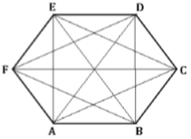Sides of hexagon: AB, BC, CD, DE, EF and FA.
Diagonals of hexagon: AC, AD, AE, BD, BE, BF, CE, CF, and DF
4.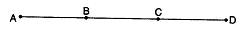{tex}\because{/tex} B is the mid-point of {tex}\overline{A C}{/tex}
∴ AB = BC …(1)
{tex}\because{/tex}C is the mid-point of {tex}\overline{B D}{/tex}
∴ BC = CD …(2)
In view of (1) and (2), we get
AB = CD.
5.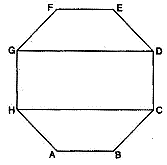1. 45°
2. 125°
3. 90°
4. ∠1 = 40°, ∠2 = 125° and ∠3 = 95°.
6. An isosceles triangle is any triangle with 2 sides that are equal in length. So every equilateral triangle is a special case of an isosceles triangle since not just 2 sides are equal, but all 3 are. But every isosceles triangle is not equilateral, because you can have 2 sides of equal length and a third side that is either longer or shorter than those 2 sides. For example, if the triangle is a right-angle triangle and the two sides that meet to make the right angle are the same length, then the 3rd side would be longer than those two.

## Chapter Wise Extra Questions for Class 6 Mathematics## Test Generator

Create Tests with your Name & Logo## Job Openings at myCBSEguide

We are hiring Subject Matter Experts and Video Content Creators for our Dwarka, New Delhi office.